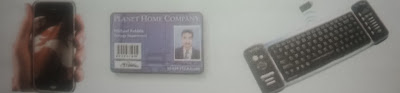# Lesson Planning of Units of Lengths

Lesson Planning of Conversion of Units of Lengths

Subject Mathematics

Students` Learning Outcomes

• Convert centimetres to millimetres and vice versa

Information for Teachers

• A millimetre is one-thousandth of a meter shortly written as “mm”.
• It is roughly thickness of our finger nail or a paperclip and the smallest measurement unit on a scale.• Centimeter is further divided into a smaller unit ‘millimetres’.
• 1 centimeter = 10 millimetres.
• Multiply the centimeter by 10 to get millimetre, as; mm = cm x 10.
• Divide the number of millimetres by 10 to get centimetres. , as
• During this lesson the teacher should consult with textbook at each level where required.

Material / Resources

Measuring tape, scale, writing board, chalk/marker, duster, prints of fee and hand, flashcards, textbook

Introduction

• Ask the students today we will know to convert centimetres to millimetre and millimetres to centimetres.
• Ask the students in which units do you think the length and thickness of your nail can be measured?
• Quote examples from daily life as; a rain gauge (instrument which is used to measure the amount of rain) which measures rain in millimetres.
• Ask the students to bring out their scales and observe the centimeter and millimetre lines.
• Tell them between any two centimetres lines there are 10 small lines represent millimetres.

Development

 Activity 1 Converting of centimetres into millimetres: Use most commonly used items so students may be able to better understand the concept of conversion of small units (e.g. cell phone, employee card, keyboard, geometry box, text books etc.)Hold your scale in your hand and point out the centimeter and millimetre lines on it.Ask the students to take out their text books and scales. Measures the lengths of these books in millimetres. Write the measurements of one student on writing board (e.g. 20 cm). Tell the students we will multiply centimetres with 10 to get millimetres, as 1 cm = 10 mm length of note book = 20 cm mm = cm x 10 length of note book in mm = 20 cm x 10 = 200 mm Conversion from millimetres to centimetres: Call one student to measure your cell phone with the help of scale in millimetres. Suppose student responded 60 mm. Convert this measurement in centimetres. Tell them we will divide millimetres with 10 to get centimetres, as; cm = mm ÷10 length of cell phone = 60 mm Length of cell phone in centimetres = 10 ÷ 60 = 6 cm.

 Activity 2 Ask the students to measure the length of their pencil in centimetres with the help of scale. Direct a student to make a table on the board and note the responses of students.Call different students and ask them to convert these measurements in ‘mm’ and write on the board.Repeat different examples for the conversion of millimetres to centimetres.

 Activity 3 Divide the class in groups and ask them to measure the following lengths in millimetres using a ruler. Height of your friend = ___. Table = _______. Note book =  _________ Geometry box = _____. Duster = ____. Ask the groups to convert the lengths into centimetres.

Sum up / Conclusion

• 1 cm can be further divided into smaller unit’s known as millimetres.
•  A Millimetres is the smallest unit of measurement shortly written as; “mm”.
• 1 cm = 10 mm
• Multiply the centimetres by 10 to get millimetres, as; mm = cm x 10.
• Divide the number of millimetres by 10 to get centimetres, as cm = mm ÷10

Assessment

• Distribute the following questions among the students and ask them to choose the best answer, as ;
• The paper clip is 3 ______ long.

(a)    Centimetres (b) kilometres

• The scale shown on ruler is in ________.

(a)    Meters   (b) centimetres

• Lead of pencil is measured in _________.

(a)    Centimetres  (b) millimetres

• Ayesha`s geometry is ______ long.

(a)    Millimetres   (b) centimetres